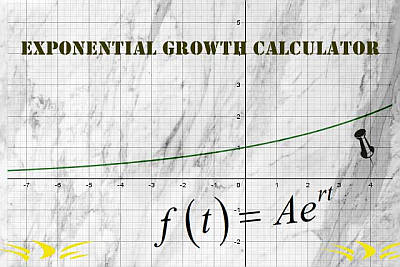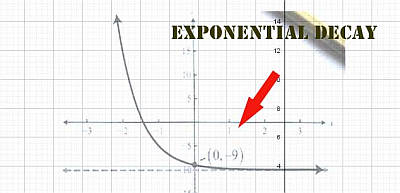# Exponential Growth Calculator

Instructions: Use this step-by-step Exponential Growth Calculator with steps to find the function that describe the exponential growth for the given parameters. You need to provide the initial value $$A_0$$, increase rate per period (which could be yearly or continuous).InitialValue $$A_0$$ (number or fraction) =Increase Rate $$r$$ (Ex. 0.04, 14%, etc) =Points to evaluate (Optional. Comma or space separated) =
Compounding Period:

## The Exponential Growth Calculator

Use this exponential growth calculator to specify a function that grows, by providing its initial value and its growth (or decay rate). In order to make a valid growth rate, provide a positive rate.

Plus, you have the option of deciding how is this rate acting, either yearly or continuously. Then you click on "Calculate" to get all the steps shown.

Exponential Growth is an algebraic behavior that has many uses in real life, from Finance to Economics, from Social Sciences to Biology. It represents a growth that is compounded every period by a certain rate (or percentage).

One way of seeing is that the rate of change is proportional to the size of the function.## Exponential Growth Formula

It is said that a function $$f(t)$$ has an exponential growth behavior if it can be expressed as:

$f(t) = A_0 (1 + r)^t$

For the above formula, $$r$$ corresponds to the growth rate, expressed as a decimal number or as a percentage (they are equivalent).

Typically, you will be provided with the compounding growth rate and the initial value $$A_0$$, but sometimes you will be provided with information about the function, and you will have to infer the parameters $$r$$ and $$A_0$$.

For the above exponential growth formula, there is a special case where the rate is compounded continuously, in which case the formula becomes

$f(t) = A_0 e^{rt}$

Typically, exponential growth functions represent money, but like we mentioned before, the can represent a variety of phenomena, such as population growth.

This type of phenomena can be seen reflected in the exponential curve, which starts relatively flat, but it rapidly increases.

## Exponential Growth Applications

You can use this exponential function calculator for different types of models, provided that you know the parameters that are required.

One typical such model involves specific populations that grow rapidly. This may happen to bacteria, insect and even human population. Usually, as populations rapidly grow, competition for resources becomes stringent and the growth stops being exponential.

Observe that this calculator will also provide you with the graph of the resulting exponential function.## How are Exponential Growth and Exponential Decay Related?

Exponential growth and exponential decay are absolutely analogous, and the main difference is that the rate $$r$$ in exponential growth is positive, and it is negative in exponential decay.

You can also can use this exponential decay calculator for the reverse but analogous exponential behavior, that corresponds to exponential decay, where the growth rate now is negative.

So, how do I know if it is growth or decay? Simple, you just look at the rate, and if it is positive, then you have growth and if it is negative you then have decay.

Both the exponential growth and decay calculator will show you all the steps, which essentially consists of solving two simultaneous equations with two unknowns

.

## Exponential Growth from Two points

Now, you may want to calculate an exponential function from two points where it is known it passes through.

But, how do you find the exponential growth rate with two points? You start with a generic exponential equation of the form $$f(t) = A_0 e^{r t}$$. This equation has two unknowns which are $$A_0$$ and $$k$$.

So by plugging two points $$(t_1, y_1)$$ and $$(t_2, y_2)$$ into $$f(t) = A_0 e^{r t}$$, you will have two equations with two unknowns, which will be solvable provided that $$t_1 \ne t_2$$, which makes sense, because we don't want two points with the same x-coordinate.